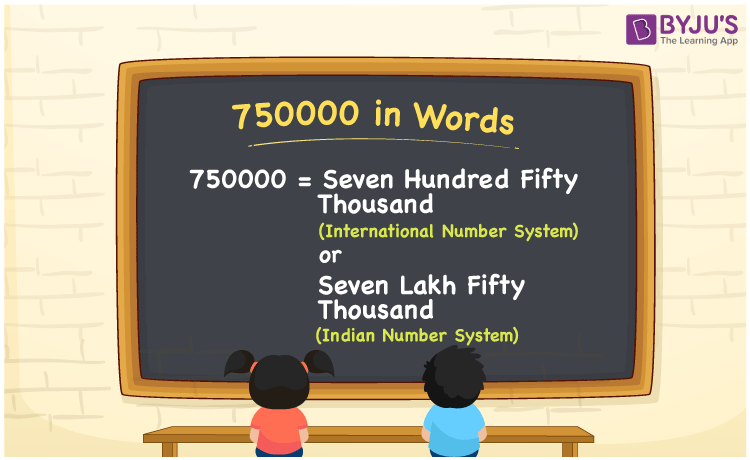# 750000 in Words

750000 in words is written as Seven Hundred Fifty Thousand (in International Number System) or Seven Lakh Fifty Thousand (Indian Number System. For example, if we need to write a cheque of Rs. 750000, then it is given by Rupees Seven Hundred Fifty Thousand. In Maths, 750000 is a counting number, that represents a value or a quantity. Learn how to represent 750000 in words, in this article.

 750000 in Words Seven Hundred Fifty Thousand (International number system) Seven Lakh Fifty Thousand (Indian Number System) Seven Hundred Fifty Thousand 750000

## 750000 in English Words## How to Write 750000 in Words?

To convert 750000 to words, we can use a place value chart, to represent the positions of digits in the given number. The number 750000 has 6 digits, thus;

 One Hundred Thousand or  One Lakh Ten thousand Thousands Hundreds Tens Ones 7 5 0 0 0 0

From the above table, we can write the number 750000 as Seven Hundred Fifty Thousand or Seven Lakh Fifty Thousand.

### Expanded Form of 750000

We can write the expanded form as:

7 x Lakhs + 5 × Ten thousand + 0 × Thousand + 0 × Hundred + 0 × Ten + 0 × One

= 7 x 100000 + 5 × 10000 + 5 × 1000 + 0 × 100 + 0 × 10 + 0 × 1

= 750000

= Seven Hundred Fifty Thousand

or

= Seven Lakh Fifty Thousand

750000 is the natural number that is succeeded by 749999 (750000 – 1) and preceded by 750001 (750000 + 1). Learn more about the number 750000 below:

• 750000 in Words – Seven Hundred Fifty Thousand/Seven Lakh Fifty Thousand
• Is 750000 an odd number? – No
• Is 750000 an even number? – Yes
• Is 750000 a perfect square number? – No
• Is 750000 a perfect cube number? – No
• Is 750000 a prime number? – No
• Is 750000 a composite number? – Yes

## Frequently Asked Questions on 750000 in words

Q1

### What is 750000 in words?

750000 in words is given by Seven Hundred Fifty Thousand or Seven Lakh Fifty Thousand.
Q2

### What is the place value of 5 in 750000?

The place value of 5 in 750000 is Ten Thousands
Q3

### How to spell 750000 in English?

750000 in English is spelt as Seven Hundred Fifty Thousand or Seven Lakh Fifty Thousand